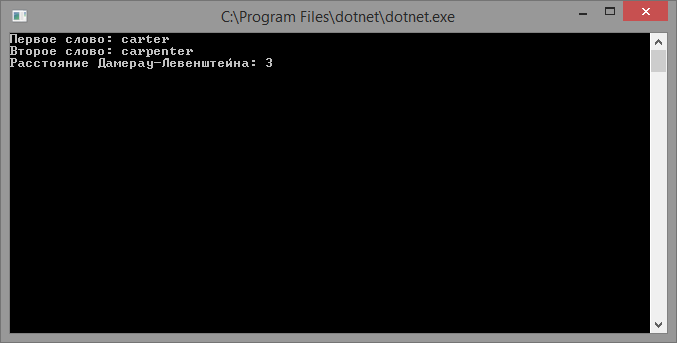# Damerau-Levenshtein distance

Updated: 15.09.2019

Damerau-Levenshtein distance is a metric for determining the distance between two lines. It can be defined as the minimum number of deletion, insertion, replacement, and transposition operations (permutation of two adjacent characters) needed to convert one line to another.

The Damerau-Levenshtein distance differs from the classical Levenshtein distance in that it includes transposition among its valid operations in addition to the three classic single-character editing operations:

• inserts;
• deletion;
• replacements.

In his work, Damerau stated that more than 80% of all spelling errors can be attributed to one of four types. Damerau’s work considered only spelling errors that could be corrected by no more than one editing operation. While the initial task was to measure the spacing between spelling errors to improve spell checking applications. The Damerau-Levenshtein distance is also used in biology to measure variations between protein sequences.

## Description of the algorithm

To search for the Damerau-Levenshtein distance, an algorithm is used in which it is necessary to fill in the D matrix with the size n + 1 by m + 1, where n and m are the lengths of the compared lines A and B according to the following rules:

• D 0,0 = 0
• D i, j = Minimum of:
• D i-1, j-1 + 0 (characters are the same)
• D i, j-1 + w delete (delete character)
• D i-1, j + w insert (insert character)
• D i-1, j-1 + w replace (character replacement)
• D i-2, j-2 + w transpose (character permutation)

w delete , w insert , w replace , w transpose - price or weight of deleting, inserting, replacing, and rearranging a character.

Moreover, D n-1, m-1 - contains the value of the Damerau-Levenshtein distance.

## Implementation of the algorithm

``````using System;

class program
{
static int Minimum(int a, int b) => a < b ? a : b;

static int Minimum(int a, int b, int c) => (a = a < b ? a : b) < c ? a : c;

static int DamerauLevenshteinDistance(string firstText, string secondText)
{
var n = firstText.Length + 1;
var m = secondText.Length + 1;
var arrayD = new int[n, m];

for (var i = 0; i < n; i++)
{
arrayD[i, 0] = i;
}

for (var j = 0; j < m; j++)
{
arrayD[0, j] = j;
}

for (var i = 1; i < n; i++)
{
for (var j = 1; j < m; j++)
{
var cost = firstText[i - 1] == secondText[j - 1] ? 0 : 1;

arrayD[i, j] = Minimum(arrayD[i - 1, j] + 1, // delete
arrayD[i, j - 1] + 1, // insert
arrayD[i - 1, j - 1] + cost); // replacement

if (i > 1 && j > 1
&& firstText[i - 1] == secondText[j - 2]
&& firstText[i - 2] == secondText[j - 1])
{
arrayD[i, j] = Minimum(arrayD[i, j],
arrayD[i - 2, j - 2] + cost); // permutation
}
}
}

return arrayD[n - 1, m - 1];
}

static void Main(string[] args)
{
Console.Write("First word:");
The result of the program: